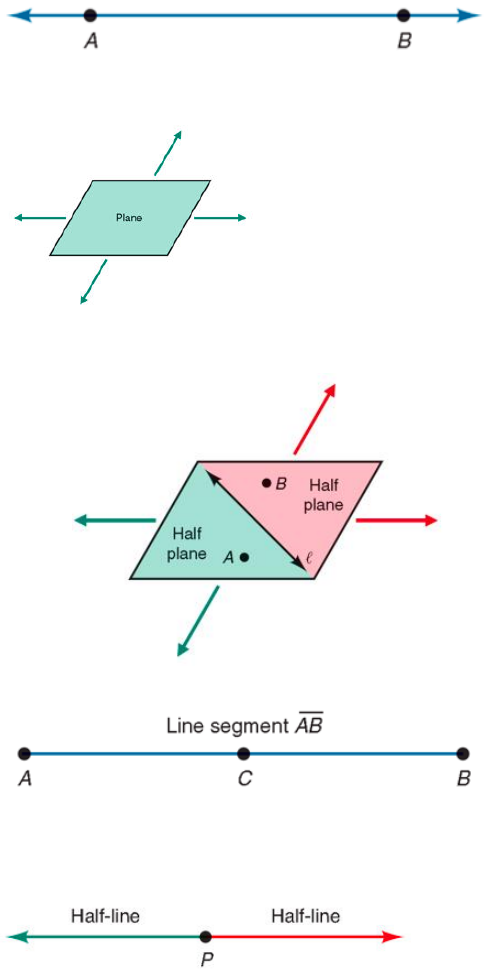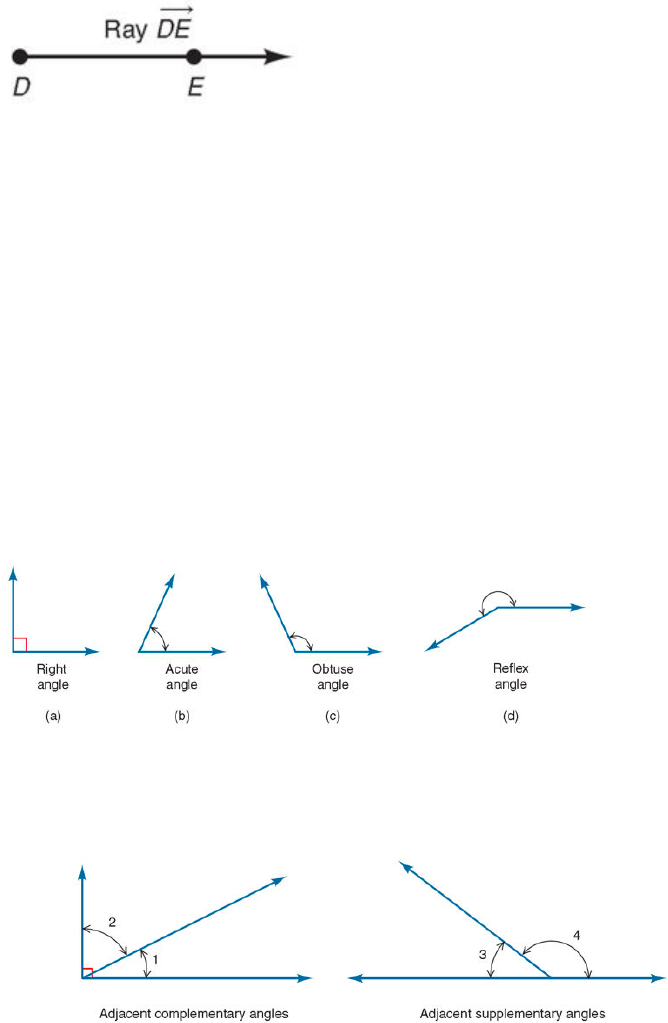# MTH 152 Lecture Notes - Lecture 10: Solar Energy Generating Systems, Deductive Reasoning, Acute And Obtuse Triangles

11 views7 pages
School
Department
Course
ProfessorMathematical Systems: consists of undefined terms, definitions, axioms, and theorems
must always be some words that are undefined (ex: “Line”)
undefined terms: To avoid this problem of circularity, certain basic
words (ex: point, line, plane)
words are then used in definitions to define other words
Axioms: statements that we assume to be true and do not try to prove
Theorems: reason we use axioms, definitions, and undefined terms are used
together with deductive reasoning to prove theorems
Line: set of points that we describe intuitively as being “straight” and extending
indefinitely in both direction
Collinear: when 2 or more points are on the same line
Plane: “flat” like the top of a table, but extending indefinitely
Half-planes: formed when line in a plane partitions the plane into 3 separate sets:
the points on the line and 2 half-planes
line segment: 2 points on a line and all the points between them
Bisection: divide line seg. into 2 parts of equal length
Midpoint: point between endpoints
Half-line: point on line separates line into 3 separate sets: the point and two half-lines
Ray: line with a single endpoint (or point of origin) that extends infinitely in one direction.
Unlock document

This preview shows pages 1-2 of the document.
Unlock all 7 pages and 3 million more documents.Angle: formed by the union of two rays, with a common endpoint
Vertex: endpoint of angle
sides of the angle: rays/line segments
Angle measurements
Degrees: method for measuring angles by dividing a circle into 360 equal parts
One degree (1°) is 1/360 of a complete turn in a circle
Minutes: Each degree can be divided into 60 equal parts
Seconds: each minute can be divided into 60 equal parts
Types of angles
right angle: If an angle has a measure of 90°
Acute angle: less than 90°
obtuse angle: more than 90° and less than 180°
straight angle: 180°
reflex angle: more than 180° and less than 360°
draw a circular arc to connect the two sides of this angle
Complementary angles: sum of two angles is 90°
Supplementary angles: sum is 180°
adjacent angles: If two angles have the same vertex, share a common side, and
do not overlap
Vertical angles (across from eachother): Nonadjacent angles formed by two
intersecting lines; have equal measure
Unlock document

This preview shows pages 1-2 of the document.
Unlock all 7 pages and 3 million more documents.